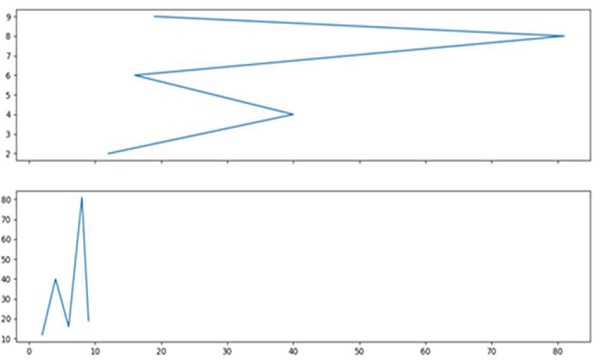# How to share x axes of two subplots after they have been created in Matplotlib?

MatplotlibServer Side ProgrammingProgramming

#### Python Data Science basics with Numpy, Pandas and Matplotlib

Most Popular

63 Lectures 6 hours

#### Data Visualization using MatPlotLib & Seaborn

11 Lectures 4 hours

#### MatPlotLib with Python

9 Lectures 2.5 hours

First, we can create two axes using the subplot method where nrows=2, ncols=1. That means, we can have two indices to plot the desired plot. We can use ax1.get_shared_x_axes().join(ax1,ax2) method for our plot.

## Steps

• Create two lists of the numbers.

• Add a subplot to the current figure, ax1, where nrows = 2, ncols = 1, and index is 1 for ax1.

• Add a subplot to the current figure, ax2, where nrows = 2, ncols = 1, and index is 2 for ax2.

• Plot x and y using points that are created in step 1.

• Using get_shared_x_axes().join(ax1, ax2), return a reference to the shared axes Grouper object for axes.

• Set X-axis tick labels of the grid, with empty list, using set_xticklabels method.

• Use plt.show() to show the figure.

## Example

import matplotlib.pyplot as plt

x = [2, 4, 6, 8, 9]
y = [12, 40, 16, 81, 19]

ax1 = plt.subplot(211)
ax2 = plt.subplot(212)

ax1.plot(y, x)
ax2.plot(x, y)

ax1.get_shared_x_axes().join(ax1, ax2)
ax1.set_xticklabels([])

plt.show()

## Output